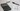# JavaScript 中的指数(幂)运算

``````// Old way
const old = Math.pow(3, 7);

// 2187
// ✅ ES7 way
const es7 = 3 ** 7;
// 2187``````

## 中缀表示法(Infix notation)

es7 引入这个语法的原因是：

`**` 相比函数而言更加简单明了，使其更为可取

## 其他语言中的指数运算

``````// Python
a ** b;

// Ruby
a ** b;

// Perl
a ** b;

// F#
a ** b;``````

## 赋值运算

``````a += b;

// Same as
// a = a + b``````

``````a **= b;

// Same as
// a = a ** b``````

## 负基数

``````// ❌ 语法错误
const wrong = (-3) ** 7;

// ✅
const correct = (-3) ** 7;``````

``const works = Math.pow(-3, 7); // 正确的``